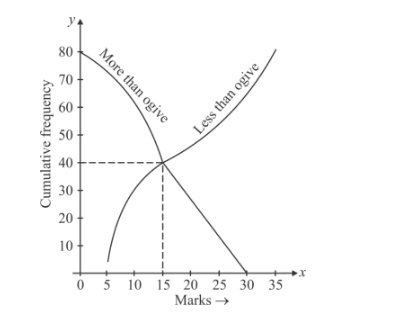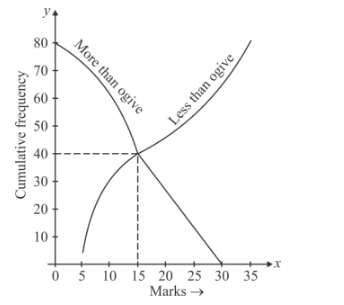# In Fig. 1, the value of the median of the data`
Question:

In Fig. 1, the value of the median of the data using the graph of less than ogive and more than ogive is

(a) 5

(b) 40

(c) 80

(d) 15Solution:

Here we have to find the median from the given graph.

The following graph is given.From the given graph we can easily see that the total cumulative frequency N = 80.

First we draw a line from the point $\frac{N}{2}=\frac{80}{2}=40$ on the less than ogive graph which is parallel to the $x$-axis and draw a line perpendicular to the $x$-axis from that point, which meet the $x$-axis at $x=$ 15 which is the desired median.

Hence the correct option is (d)